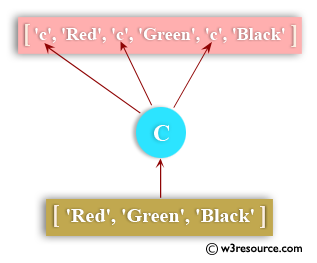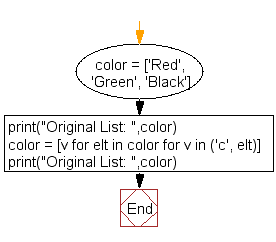﻿ Python: Insert an element before each element of a list - w3resource# Python: Insert an element before each element of a list

## Python List: Exercise - 47 with Solution

Write a Python program to insert an element before each element of a list.Sample Solution:-

Python Code:

``````color = ['Red', 'Green', 'Black']
print("Original List: ",color)
color = [v for elt in color for v in ('c', elt)]
print("Original List: ",color)
```
```

Sample Output:

```Original List:  ['Red', 'Green', 'Black']
Original List:  ['c', 'Red', 'c', 'Green', 'c', 'Black']
```

Flowchart:## Visualize Python code execution:

The following tool visualize what the computer is doing step-by-step as it executes the said program:

Python Code Editor:

Have another way to solve this solution? Contribute your code (and comments) through Disqus.

What is the difficulty level of this exercise?

Test your Python skills with w3resource's quiz

﻿

## Python: Tips of the Day

Floor Division:

When we speak of division we normally mean (/) float division operator, this will give a precise result in float format with decimals.

For a rounded integer result there is (//) floor division operator in Python. Floor division will only give integer results that are round numbers.

```print(1000 // 300)
print(1000 / 300)```

Output:

```3
3.3333333333333335```